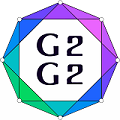#Graphs and Groups, Geometries and GAP (G2G2) Summer School - External Satellite Conference of 8ECM

27 June 2021 to 3 July 2021
Rogla, Slovenia
UTC timezone

## On finite non-solvable 4-primary groups without elements of order 6

Not scheduled
20m
Rogla, Slovenia

#### Rogla, Slovenia

Hotel Planja, Rogla 1, 3214 Zreče, Slovenia
Oral presentation

### Speaker

Mr Nikolai Minigulov (Krasovskii Institute of Mathematics and Mechanics UB RAS)

### Description

We use mainly standard notation and terminology (see ).

Let $G$ be a finite group. Denote by $\pi(G)$ the set of all prime divisors of the order of $G$. If $|\pi(G)| = n$, then $G$ is called $n$-primary.

The Gruenberg--Kegel graph (prime graph) $\Gamma(G)$ of $G$ is a graph with the vertex set $\pi(G)$, in which two distinct vertices $p$ and $q$ are adjacent if and only if there exists an element of order $pq$ in $G$.

In 2012–2013, A.S. Kondrat'ev described finite groups having the same Gruenberg-Kegel graph as the groups $Aut(J_2)$  and $A_{10}$ , respectively. The Gruenberg--Kegel graphs of these groups are isomorphic as abstract graphs.

We establish a more general problem: to describe finite groups whose Gruenberg-Kegel graphs are isomorphic as abstract graphs to the graph $\Gamma(A_{10})$.

As a part of the solution of this problem, we proved in  that if $G$ is a finite non-solvable group and the graph $\Gamma(G)$ as abstract graph is isomorphic to the graph $\Gamma(A_{10})$, then the quotient group $G/S(G)$ (where $S(G)$ is the solvable radical of $G$) is almost simple, and classified all finite almost simple groups whose the Gruenberg-Kegel graphs as abstract graphs are isomorphic to subgraphs of $\Gamma(A_{10})$.

Let $G$ be a finite non-solvable group, and the graph $\Gamma(G)$ as abstract graph is isomorphic to the graph $\Gamma(A_{10})$. Then the graph $\Gamma(G)$ has the following form (see attachment pdf), where $p$, $q$, $r$, and $s$ are pairwise distinct primes.

In , we described such finite non-solvable groups $G$ when 3 does not divide $|G|$.

In this work, we prove the following theorem.

Theorem. Let $G$ be a finite non-solvable group, $\overline{G}\cong G/S(G)$ and $\Gamma(G)$ as abstract graph is isomorphic to $\Gamma{(A_{10})}$. If $3$ divides of $|G|$ and $G$ has no elements of order $6$, then one of the following statements holds:

$(1)$ $S=O_{2',2}(G)$, $O(G)=O_p(G)$, $q=2$, $S/O(G)$ is an elementary abelian $2$-group, $\overline{G}\cong{L_2(2^n)}$ and one of the following statements holds:

$(1a)$ $n=4$, $p=17$ and $\{r,s\}=\{3,5\}$;

$(1b)$ $n$ is prime, $n\ge5$, $p=2^n-1$, and $\{r,s\}=\{3,(2^n-1)/3\}$, the group $S/O(G)$ either is trivial or as $\overline{G}$-module is isomorphic to a direct sum of natural $GF(2^n)\overline{G}$-modules;

$(2)$ $S=O_p(G)$, $q=2$, $\overline{G}\cong L_2(p)$, $p\ge31$, $p\equiv\varepsilon5(mod$ $12)$, $\varepsilon\in\{+,-\}$, $p-\varepsilon1=2^k$, and $3\in\{r,s\}=\pi((t+\varepsilon1)/2)$;

$(3)$ $S=O_p(G)$, $q=3$, and one of the following statements holds:

$(3a)$ $\overline{G}\cong PGL_2(9)$, $p>5$, and $\{r,s\}=\{2,5\}$;

$(3b)$ $\overline{G}\cong L_2(81)$, $PGL_2(81)$ or $L_2(81).2_3$, $p=41$, and $\{r,s\}=\{2,5\}$;

$(3c)$ $\overline{G}\cong L_2(3^n)$ or $PGL_2(3^n)$, $n$ is an odd prime, $p=(3^n-1)/2$, and $\{r,s\}=\pi(3^n+1)$.

Each of the statements $(1)$--$(3)$ of the theorem is realized.

In the proof of Theorem, we use the classification of the finite non-solvable groups without elements of order $6$ from (Theorem 2, ).

Acknowledgments. The work is supported by Russian Science Foundation (project 19-71-10067).

References:
 J. H. Conway, R. T. Curtis, S. P. Norton, R. A. Parker, R. A. Wilson, Atlas of finite groups, Clarendon Press, Oxford (1985).
 A. S. Kondrat'ev, Finite groups with prime graph as in the group $Aut(J_2)$. Proc. Steklov Inst. Math. 283(1) (2013), 78--85.
 A. S. Kondrat'ev, Finite groups that have the same prime graph as the group $A_{10}$. Proc. Steklov Inst. Math. 285(1) (2014), 99--107.
 A. S. Kondrat'ev, N. A. Minigulov, Finite almost simple groups whose Gruenberg--Kegel graphs as abstract graphs are isomorphic to subgraphs of the Gruenberg--Kegel graph of the alternating group $A_{10}$. Siberian Electr. Math. Rep. 15 (2018), 1378--1382.
 A. S. Kondrat'ev, N. A. Minigulov, On finite non-solvable $4$-primary $3'$-groups, in: Algebra, number theory and mathematical modeling of dynamical systems: abstracts of the international conference devoted to the $70$th anniversary of A.Kh. Zhurtov, KBSU, Nal'chik, 2019, 56 (in Russian).
 A. S. Kondrat'ev, N. A. Minigulov, Finite groups without elements of order six. Math. Notes 104:5 (2018), 696--701.

### Primary authors

Prof. Anatoly Kondrat'ev (Krasovskii Institute of Mathematics and Mechanics UB RAS) Mr Nikolai Minigulov (Krasovskii Institute of Mathematics and Mechanics UB RAS)